Question

# The diameter of 0–gauge copper wire is 8.252 mm. Find the resistance of a 5.00–km length...

The diameter of 0–gauge copper wire is 8.252 mm. Find the resistance of a 5.00–km length of such wire used for power transmission.

Use 1.719e-08 as the resistivity for Cu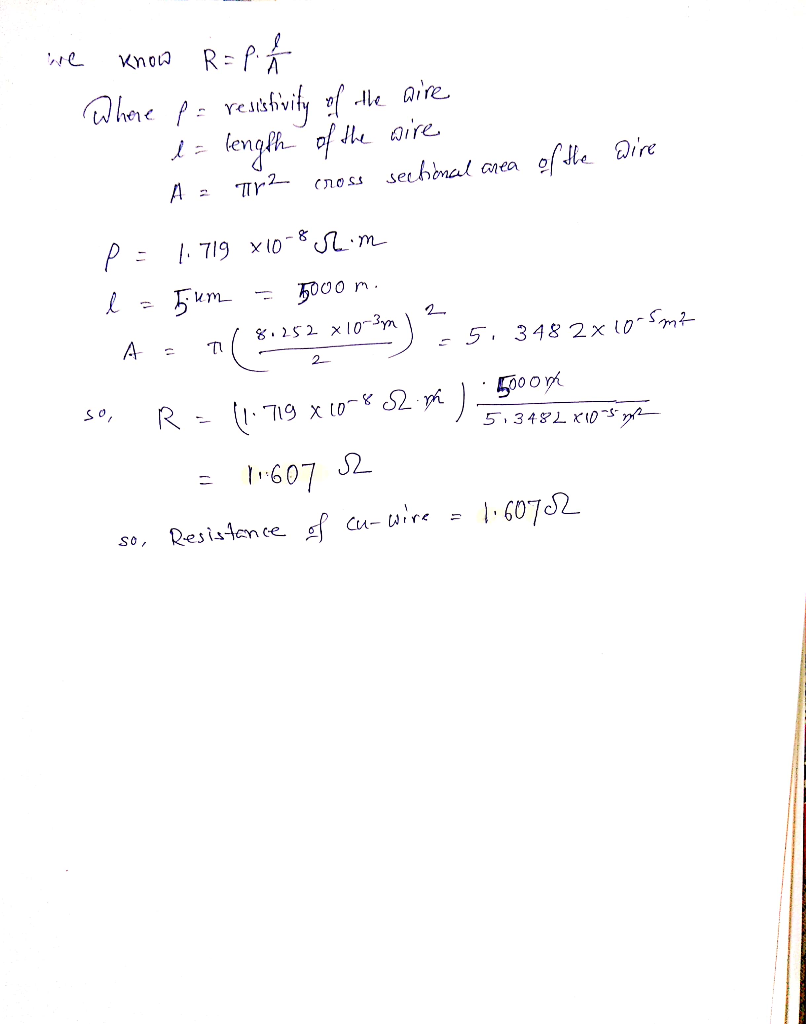#### Earn Coins

Coins can be redeemed for fabulous gifts.

Similar Homework Help Questions
• ### The diameter of Four Gauge copper wire is 5.189 mm. Calculate the resistance (in Q) of...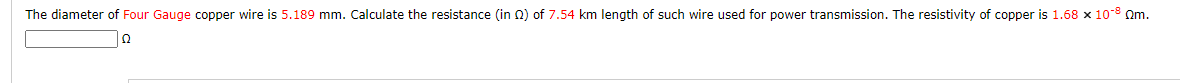The diameter of Four Gauge copper wire is 5.189 mm. Calculate the resistance (in Q) of 7.54 km length of such wire used for power transmission. The resistivity of copper is 1.68 x 10-9 Om.

• ### The diameter of Twelve Gauge copper wire is 2.052 mm. Calculate the resistance (in 2) of...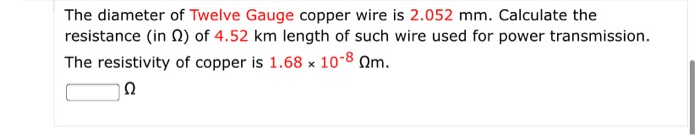The diameter of Twelve Gauge copper wire is 2.052 mm. Calculate the resistance (in 2) of 4.52 km length of such wire used for power transmission. The resistivity of copper is 1.68 x 10-8 Am. Ω

• ### What is the resistance of a 2.9-m length of copper wire 1.8 mm in diameter? The...

What is the resistance of a 2.9-m length of copper wire 1.8 mm in diameter? The resistivity of copper is 1.68×10−8Ω⋅m.

• ### What is the resistance of a 5.9-m length of copper wire 1.2 mm in diameter? The...

What is the resistance of a 5.9-m length of copper wire 1.2 mm in diameter? The resistivity of copper is 1.68×10−8Ω⋅m.

• ### What is the resistance of a 4.9-m length of copper wire 1.9 mm in diameter? The...

What is the resistance of a 4.9-m length of copper wire 1.9 mm in diameter? The resistivity of copper is 1.68×10−8Ω⋅m.

• ### A length of 20-gauge copper wire (of diameter 0.8118 mm) is formed into a circular loop...

A length of 20-gauge copper wire (of diameter 0.8118 mm) is formed into a circular loop with a radius of 26.0 cm. A magnetic field perpendicular to the plane of the loop increases from zero to 18.0 mT in 0.24 s. Find the average electrical power dissipated in the process.

• ### 2. A length of 20-gauge copper wire (of diameter 0.8118 mm) is formed into a circular...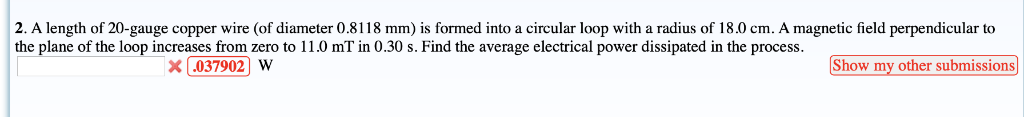2. A length of 20-gauge copper wire (of diameter 0.8118 mm) is formed into a circular loop with a radius of 18.0 cm. A magnetic field perpendicular to the plane of the loop increases from zero to 11.0 mT in 0.30 s. Find the average electrical power dissipated in the process. X.037902) W Show my other submissions

• ### 1) 10 meters of 12-gauge copper wire (2 mm diameter) is used to carry 20 A...

1) 10 meters of 12-gauge copper wire (2 mm diameter) is used to carry 20 A DC current. a]- Calculate the resistance of the wire, b]- the voltage drop, ,,,,<<<< Please step by step , I want to learn >>>.... c]- the power loss ? = ??, and d]- the magnitude of J, E in the wire

• ### How much is the resistance of 50 ft if household 14 gauge Cu wire (1.63 mm...

How much is the resistance of 50 ft if household 14 gauge Cu wire (1.63 mm in diameter)? Resistivity of Cu is 1.70 *10^-8 Ohm m, if the current 15 A is flowing in this wire, then how much is the voltage drop on it?

• ### Wire is classified according to its diameter using the American Wire Gauge (AWG) scale. AWG 19...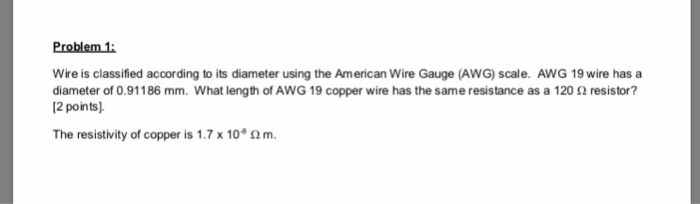Wire is classified according to its diameter using the American Wire Gauge (AWG) scale. AWG 19 wire has a diameter of 0.911 86 mm. what length of AWG 19 copper wire has the same resistance as a 120 Ω resistor? 2 points) The resistivity of copper is 1.7 x 104 Ω m.